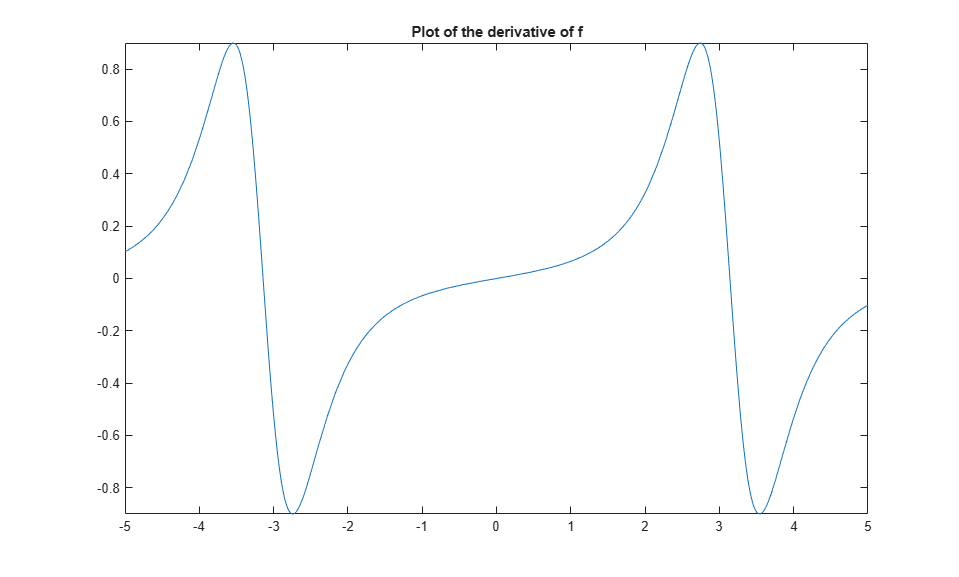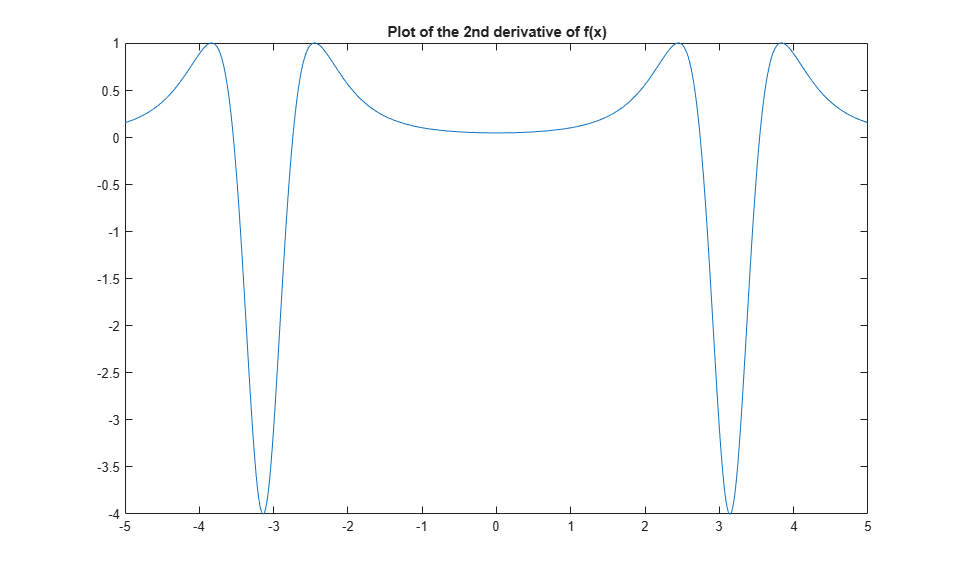Learn Calculus Using Symbolic Math Toolbox

Learn calculus and applied mathematics using the Symbolic Math Toolbox™. This example shows the introductory functions fplot, diff, and int.

To manipulate a variable, create an object of type syms.

disp('Create a symbolic variable for x')
disp('>> syms x')
syms x
Create a symbolic variable for x
>> syms x

After a symbolic variable is defined, you can build and visualize functions with fplot.

disp('Build the function f(x) and plot it')
disp('>> f(x) = 1/(5+4*cos(x))')
disp('>> fplot(f)')
f(x) = 1/(5+4*cos(x))
figure;
fplot(f)
title('Plot of f(x)')
Build the function f(x) and plot it
>> f(x) = 1/(5+4*cos(x))
>> fplot(f)

f(x) =

1/(4*cos(x) + 5)Evaluate the function at x = pi/2 using math notation.

disp('Evaluate f(x) at x = pi/2')
disp('>> f(pi/2)')
f(pi/2)
Evaluate f(x) at x = pi/2
>> f(pi/2)

ans =

1/5

Many functions can work with symbolic variables. For example, the function diff differentiates a function.

disp('Differentiate f(x) and plot the result')
disp('>> f1 = diff(f)')
disp('>> fplot(f1)')
f1 = diff(f)
figure;
fplot(f1)
title("Plot of the derivative of f")
Differentiate f(x) and plot the result
>> f1 = diff(f)
>> fplot(f1)

f1(x) =

(4*sin(x))/(4*cos(x) + 5)^2The diff function can also find the Nth derivative. The next examples shows the second derivative.

disp('Compute the second derivative of f(x) and plot it')
disp('>> f2 = diff(f,2)')
disp('>> fplot(f2)')
f2 = diff(f,2)
figure;
fplot(f2)
title("Plot of the 2nd derivative of f(x)")
Compute the second derivative of f(x) and plot it
>> f2 = diff(f,2)
>> fplot(f2)

f2(x) =

(4*cos(x))/(4*cos(x) + 5)^2 + (32*sin(x)^2)/(4*cos(x) + 5)^3The int function integrates functions of symbolic variables. The following example shows an attempt to retrieve the original function by integrating the second derivative twice.

disp('Retrieve the original function by integrating the second derivative twice. Plot the result.')
disp('>> g = int(int(f2))')
disp('>> fplot(g)')
g = int(int(f2))
figure;
fplot(g)
title("Plot of int(int(f2))")
Retrieve the original function by integrating the second derivative twice. Plot the result.
>> g = int(int(f2))
>> fplot(g)

g(x) =

-8/(tan(x/2)^2 + 9)Initially, the plots for f and g look the same. However, their formulas and the y-axis ranges are different.

disp('Observe the formulas and ranges on the y-axis when comparing f and g')
disp('>> subplot(1,2,1)')
disp('>> fplot(f)')
disp('>> subplot(1,2,2)')
disp('>> fplot(g)')
disp(' ')
figure;
subplot(1,2,1)
fplot(f)
title("Plot of f")
subplot(1,2,2)
fplot(g)
title("Plot of g")
Observe the formulas and ranges on the y-axis when comparing f and g
>> subplot(1,2,1)
>> fplot(f)
>> subplot(1,2,2)
>> fplot(g)The value e is the difference between f and g. It has a complicated formula, but its graph looks like a constant.

disp('Compute the difference between f and g')
disp('>> e = f - g')
e = f - g
Compute the difference between f and g
>> e = f - g

e(x) =

8/(tan(x/2)^2 + 9) + 1/(4*cos(x) + 5)

To show that the difference truly is a constant, simplify the equation.

disp('Simplify the equation to show that the difference between f and g is constant')
disp('>> simplify(e)')
e = simplify(e)
Simplify the equation to show that the difference between f and g is constant
>> simplify(e)

e(x) =

1